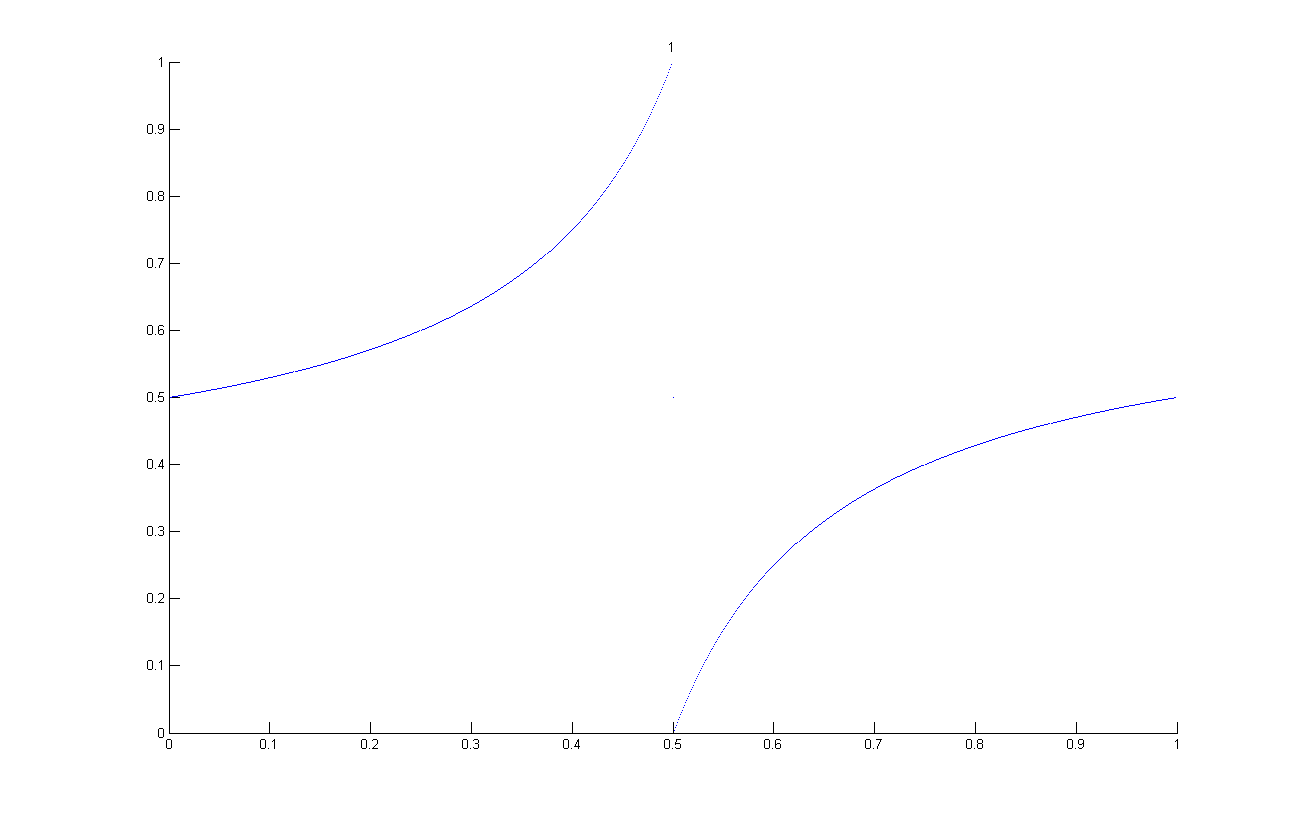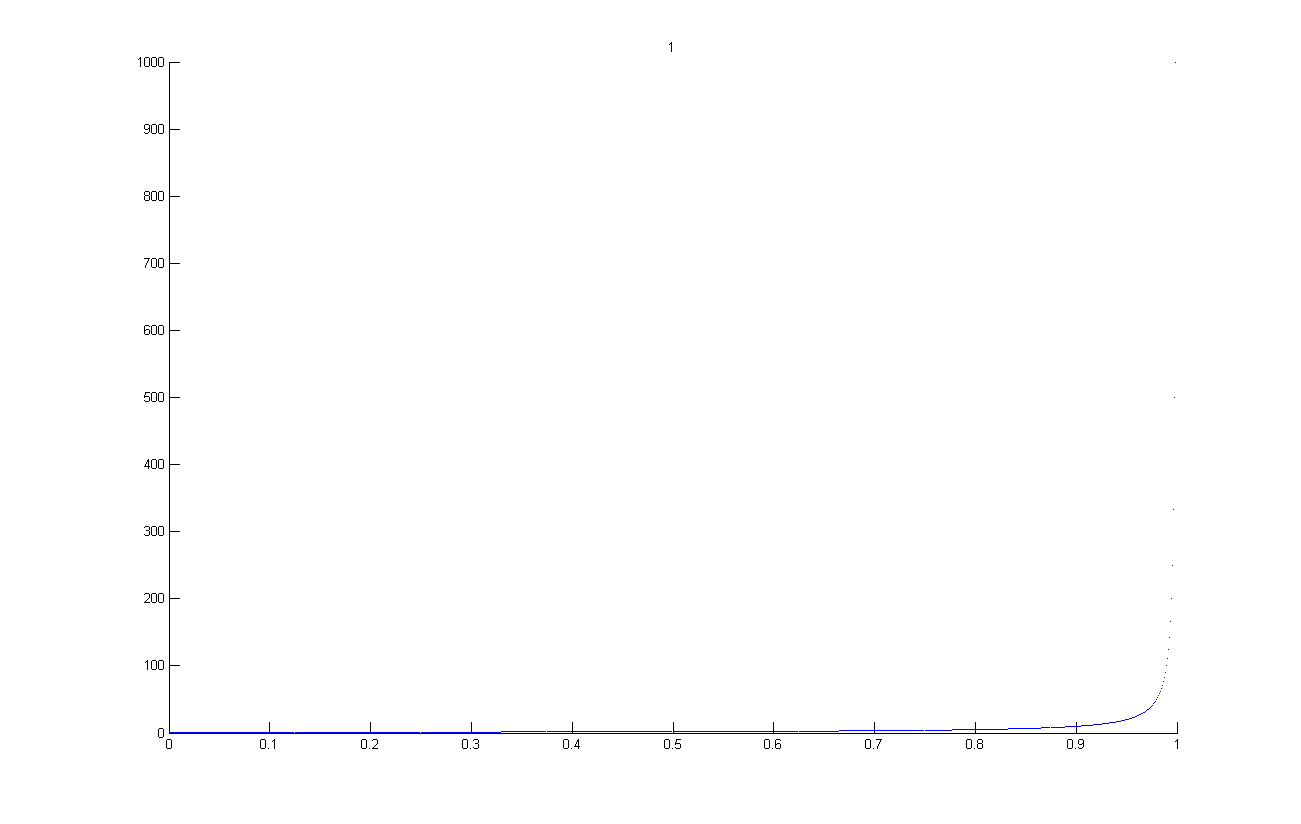## Source Codes

The matlab and python functions below both expect a single argument consisting of the continued fraction expansion, represented as a list of positive integers, of the number for which the jimm transformation will be computed. Both functions return the continued fraction expansion of the result of transformation similarly represented as a list of positive integers. All code on this page is available under GPLv3 license as expressed below.

``` This program is free software: you can redistribute it and/or modify it under the terms of the GNU General Public License as published by the Free Software Foundation, either version 3 of the License, or (at your option) any later version. This program is distributed in the hope that it will be useful, but WITHOUT ANY WARRANTY; without even the implied warranty of MERCHANTABILITY or FITNESS FOR A PARTICULAR PURPOSE. See the GNU General Public License for more details. You should have received a copy of the GNU General Public License along with this program. If not, see http://www.gnu.org/licenses/. ```

 Matlab Code Python Code ```% Copyright 2014 Muhammed Uludağ, Hakan Ayral function t = jimm(q) t = ones(1,double( q(1))-1); if(q(1)==0) t=; acc=0; else acc = 1; end for x=2:length(q) if(q(x)==1) acc=acc+1; else t = [t acc+1 ones(1,double(q(x))-2)]; acc = 1; end end if acc > 0 t = [t acc]; end ``` ```# Copyright 2014 Muhammed Uludağ, Hakan Ayral def jimm(q): if q == 0: t =  acc = 0 else: acc = 1 t =  * (q-1) for x in xrange(1,len(q)): if q[x] == 1: acc=acc+1 else: t = t+[acc+1]+ *(q[x]-2) acc = 1 if acc > 0: t = t + [acc] return t ```

## Usage Example

Pass the continued fraction representation of the number for which you want the jimm transform to be computed as a list of integers to the function.

```>> jimm([3, 7, 15, 1]) ans = 1 1 2 1 1 1 1 1 2 1 1 1 1 1 1 1 1 1 1 1 1 1 2 ```

## Some graphics

• Twist
• Jimm transformation can be represented as twisting every vertex (with one exception) of the farey graph. Twisting a node of a tree is defined such that left and right child nodes of twisted node switch places and all their respective child nodes have their horizontal direction reversed. Animated plot below show identity function y=x getting transformed by twisting all nodes on successive levels of farey tree in a breadth first manner. (please click to enlarge the animation)• Shuffle
• Another action on farey tree nodes is shuffling. Shuffling a node is defined as switching the places of left and right sub-trees of the shuffled node while keeping the order in the sub-trees intact. Animated plot below show the identity function y=x getting transformed by shuffling all nodes on successive levels of farey tree in a breadth first manner.(please click to enlarge the animation)• Shuffle 2
• Farey tree can be defined for (0,1) interval by starting with sequence [0/1,1/1], or for (0,oo) interval by starting with sequence [0/1,1/0]. Animated plot below shows the identity function y=x getting transformed by shuffling all nodes of farey tree covering (0,oo) interval for increasing number of levels in a breadth first manner.(please click to enlarge the animation)## Concepts similar to Jimm in Engineering

Digital communications implement some coding techniques to represent a data, and some signal conditioning transforms to obtain some desired properties on these representations. In analog communication when a carrier wave is used to transmit the data, as in the case of RF communications, a modulation of the carrier wave can be used to encode data; a sinusoidal carrier wave can be modulated in terms of its frequency, amplitude or phase. Be it RF, light, sound or electrical signals, the natural media used to transmit the digital information being analog, some discretizing mapping from digitally represented information to analog states is necessary.

Phase shift keying (PSK) is a modulation method to represent data in terms of changes of a sinusoidal carrier wave. When a modulation method assumes discrete values on modulated attribute of carrier wave, as in the case of digital communication, it is called "keying", hence the name phase shift keying. Binary PSK assumes two distinct states of 180 degrees separated phases with equal amplitude and frequency; simplest binary to BPSK mapping is to represent a 0 bit in digital as a specific phase and, a 1 bit as the inverted phase. . All signal are subject to mostly arbitrary phase shift during transmission (except in very controlled media like length balanced wires or PCB traces), unless the source and the receiver has another information source to decide which of two phases is the neutral one (i.e. 0 bit), PSK modulation suffers from what's called phase ambiguity. Phase ambiguity can be addressed by supplying a non-modulated reference signal to compare the phase against (like a clock signal in digital electronics) which is not always practical; or by use of another transform on binary data called differential encoding. If a digital data is differentially encoded before PSK modulation, the each bit is not mapped to a specific phase of PSK states, but it is mapped to a specific change in phase. A differentialy encoded data has the property called polarity insensitivity, which resolves the phase ambiguity issue (i.e. phone lines work no matter in which order the two copper wires are connected). Differential Manchester Encoding is a well known example of differential encoding which is used as the default encoding scheme of some versions of Ethernet (IEEE 802.3) computer network communications. Manchester encoding can also be thought of a digital counterpart of BPSK, it uses the digital clock signal to modulate the data signal via XOR operation; the resulting signal is same as the clock signal while the data bit (which is optionally differentially encoded before) is 0, and the inverse of clock signal if data bit is 1.

Jimm transformation of a number can be thought of being similar to BPSK; a number represented as zero and ones encoding the path on the farey tree, can be transformed using XOR operation to a modulation with a series of (01)n = 010101... which serves like a carrier signal or digital clock. We face the same phase ambiguity issue as in the case of BPSK, but in this case the problem can be solved by means of convention, such as by mapping the left turns to 0 and right turns to 1, and not the otherway, for farey tree encoding, and the carrier signal to be (01)n and not to (10)n thus fixing the neural phase to be carrier series starting with 0. There is no invariant signal (number) under this transformation, as carrier signal contains 1s and XORing with 1 is equivalent to negation which is an unary operator with no fixed point; but the signal (number) closest to be minimally changing under this transformation is (0011)n giving (0011)n XOR (01)n = 011(0011)n which is the same signal 180 degrees out of phase, and the numbers related to (0011)n and 011(0011)n are respectively 1+sqrt(2) and sqrt(2).

## Effect of Jimm on binary quadratic forms and their ideal class

Jimm is an involution which maps quadratic irrationals to other quadratic irrationals; hence a natural question that arises is how it affects the quadratic fields and the class group. On the link below you can find the list of binary quadratic forms by their discriminants (delta1) and the discriminants of the jimm counterpart (delta2), along with length (k) of the çark* the form belongs to, and the matrice representation of both forms (m1 is the main form related to delta1, m2 is the jimm of m1 related to delta2).

List of discriminants and matrices for quadratic fields and their jimm counter part. (tab delimited text file)